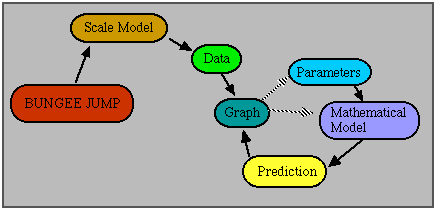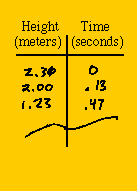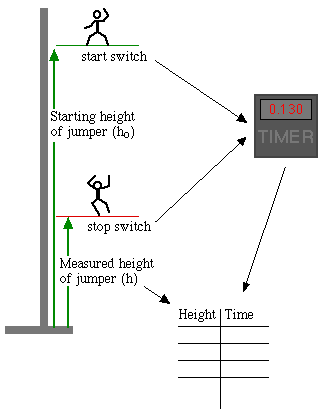##Hands On Physics

### Background: Where we are going.

Later in this unit, we are going to create a mathematical model of the bungee jump. To see whether the model is correct, we need to compare the model to real data from the scale model. Once we have confidence in our mathematical model, we can apply the model to a real jump. Here is the structure of our work:Figure P3
Structure of Work

The parameters are values that apply to a particular jump, such as the mass of the jumper and the stiffness of the elastic. When these values are supplied, the mathematical model generates a prediction. We will compare the prediction and data on a graph. Then we can change the parameters or the model until the prediction and data agree. What form does the prediction take? The model tells us where the jumper will be at any time. In mathematical terms, it predicts pairs of numbers of the form (y,t) for all any value of t, the time. Here, y is the height of the jumper above the ground. To compare the prediction to reality, you need data in the form (y,t) for several times. This means that you need to know how high the jumper is above the ground for different values of t.

Taking measurements is central to science. Recording the measurements (data) can be done in the form of a table like this:Figure P4
Data Table

We will say that t=0 is when the jumper starts. In this example, the jumper started 2.31 m above the ground.Figure P5
Timing the Jump

We start a timer when the mass just starts dropping and stop it when it crosses a height you select. The time reported by the timer is one value of t, and the height of the mass when it stopped the timer is the corresponding value of y. We then move the bottom (off) switch lower on the tower to get different values for y and t.